# A Base-40 Number-line Representation of Musical Pitch Notation

## Abstract

A base-40 number line representation of pitch notation is suggested which makes it possible to incorporate information on pitch in one number, where the difference between two pitch numbers correctly determines the notated interval name between the corresponding pitches. A general theory of 1:1 representation of pitch notation, taking into consideration the invariance of musical intervals, is suggested. The relations between the representation system suggested in the paper and other systems of pitch representation (Zimmerman's system, Clements' system) are discussed.

## 1. Introduction

Common musical notation of pitch requires two elements: (1) the letter name of the note together with its octave range, and (2) the accidentals which are applied to that note. From these elements a third attribute of musical pitch, which I call absolute pitch, may be calculated. One can think of absolute pitch as a combination of pitch class and octave range.

This study deals with pitch notation per se, not the tuning of pitches. The discussion below and the representation system which will be suggested is completely independent of a particular tuning. In principle, the approach described can be applied to any of the various tuning systems which have common musical notation as their base. The distinction is essential, since many different tuning systems, all using the same musical notation, have been devised.

Representation of musical pitch by absolute pitch alone, as is done in the MIDI protocol and in other sound applications, is not a complete representation of the pitch's notation and its attributes. For instance, C#4 and Db4 have the same absolute pitch, but they have different note names and different accidentals. This difference becomes apparent when we try to print the note or when we seek to calculate musical intervals. The interval between C#4 and Bb4 is a diminished seventh, whereas the interval between Db4 and Bb4 is a major sixth (Ex. 1).

Example 1While these intervals are of the same absolute size, they have very different harmonic functions and serve quite different purposes in structural harmony and counterpoint. In the present paper, I will try to be consistent in referring to pitch by a letter name, followed by any accidentals, followed by a number indicating the octave range of the pitch. C4, for example is Middle C, and C3 is the pitch an octave below that.
```
```

## 2. The problem of 1:1 pitch-mapping

Musical pitch may be accurately represented in the computer using the system of pitch representation mentioned above. This system goes back many years in the literature. For example, it is used in full-text databases of music at the Center for Computer Assisted Research in the Humanities (CCARH) in California, because it is easy to read and edit, and because it is not context-sensitive. This system is, however, not good for data processing: the computation of musical intervals from such ASCII encodings of pitch requires several processing steps and, depending on how one does it, a number of alternative computational paths.

Several numerical systems have been proposed for representing pitch notation in the computer. The most successful systems to be proposed thus far have been those which recognize the two-dimensional nature of pitch notation, i.e., that the unambiguous representation of pitch notation requires two independent parameters. Norbert Böker-Heil and Alexander Brinkman independently have proposed systems based on the parameters of note name and absolute pitch or pitch class. Each system includes simple algorithms for the computation of accidentals and musical interval sizes. From the standpoint of data storage and retrieval, however, the representation of musical pitch by a single numerical parameter would be superior to any system using two parameters. While any single-parameter system will have inherent ambiguities, it can be shown that for any finite set of pitch names, a single-parameter system can be constructed which is unambiguous over that set.

The construction of a single-parameter representation system, or encoding scheme, i.e., the mapping of all musically relevant pitches onto the number line, is easily accomplished. For example, we could assign the numbers 10, 20, 30, ... to the pitch names C, D, E, etc., within an octave, and then increment the basic number for each sharp, or decrement the number for each flat attached to the pitch (Ex. 2).

Example 2 A Number-line Representation of Pitch.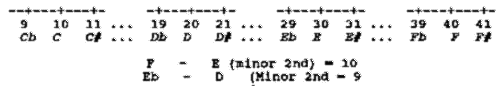The numerical size of the minor 2nd depends upon the end points.

The problem with this representation and with others constructed in a similar manner is that the calculation of musical intervals by simple subtraction of the lower number from the higher number yields different results for the same interval, depending upon which pitches are used in the calculation. In the above example, the minor second between E4 and F4 would have a value of 10, whereas the minor second between D4 and Eb4 would have the value 9. We say in this case that the interval is variant under transformation.

In constructing a mapping of pitch notation onto the number line, what we should like to have is a system for which musical intervals are invariant under transformation. It would also be convenient if each of the 24 common musical intervals within the octave had a unique numerical value.

A third condition might be to require that each of the 35 common musical pitches within the octave (comprising seven note names with accidentals from double flat to double sharp) be uniquely represented by a number. It turns out that there are several classes of solutions to the problem of constructing such a representation system, but before presenting a general theory of interval-invariant, number-line representations, I should like to propose one such solution based on an octave size of 40. This system has been in use for several years at CCARH in programs for data entry, for music printing, and for testing high-speed search algorithms in musical databases (Ex. 3).

Simple experimentation with various pitches and intervals will verify that the conditions set forth above are satisfied by this system.

```
```

## 3. Pitch representation with the base-40 system

It is instructive to present here a simple example of a "real life" musical research question which might arise in computer analysis of music. This example will illustrate how the base-40 system can be used to search for melodic intervals as well as to show why the representation of pitch by absolute pitch alone is insufficient for this purpose. The question involves the search for the melodic interval of a diminished fourth in the music of J. S. Bach. This interval is relatively uncommon in Bach's music, and it might well be of interest to study Bach's use of this interval in the context of other musical and textual elements.

Example 3In terms of absolute size, the diminished fourth encompasses the same number of half steps as a major third, namely, four half steps. For this reason, the MIDI representation of the musical passage below (Ex. 4) does not allow us to distinguish between the first melodic interval, a falling major third, and the second melodic interval, a falling diminished fourth.

Example 4: "Seufzer, Tränen, Kummer, Not" from Cantata 21, Ich hatte viel Bekümmernis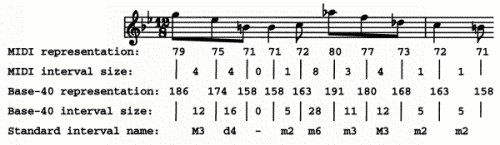The base-40 system, on the other hand, clearly differentiates between the two intervals. The first interval (186 - 174 = 12) is a major third (see "computation of intervals" in Example 3), whereas the second interval (174 - 158 = 16) is a diminished fourth.

The interesting question to ask concerning the base-40 system is what is the essential element of this system which makes it interval-invariant, and how might this element be used to construct other similar systems. The answer to this question lies in looking at the simplest case below, matched against the twelve pitches of the octave (Ex. 5).

Example 5: zero-th order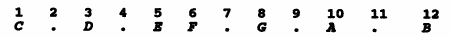Notice that where the interval between two letters is a whole step, there is an unassigned number between the letters. Notice also that intervals whose end points lie on the letters are invariant, i.e., a minor second is always 1, a major second is always 2, etc. This is a trivial system, but it does serve to establish the pattern. Using the same method of skipping a number between whole step letters, we may construct a system which includes single sharps and flats as follows (Ex. 6).

Example 6: first order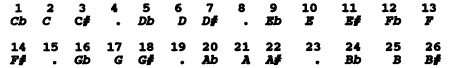```
```

## 4. The problem of interval-invariancy

Experimentation will verify that the system suggested above is interval-invariant. Let us call this system a first-order representation, since it unambiguously represents pitches with single accidentals. Using the skipping rule described above, we can construct interval-invariant, number-line representation systems of higher orders. Each successively higher order will require an additional 14 numbers for each octave. The second-order system (presented above) requires 40 numbers per octave; the third-order system will require 54 numbers; the fourth-order systems of arbitrarily high order means that from a practical standpoint, we have solved the problem of mapping arbitrarily complex pitches onto an interval-invariant, number-line system.

The subject of interval-invariant, number-line representations could be closed at this point, and indeed the present author would have been satisfied with the practical solution presented above had he not subsequently encountered in the literature two quite different systems, one by Franklin B. Zimmerman, the other by Peter J. Clements, both of which possessed the property of interval-invariance, but neither of which had the underlying structure of the base-40 system. Clearly, a more theoretical approach to this subject was needed in order thoroughly to understand this important property.

The system devised by Zimmerman appeared in his 1975 melodic index of Purcell's complete works. To my knowledge, Zimmerman was the first to devise and use an interval-invariant, number-line system for representing musical pitch. For purposes of discussion, Zimmerman's system is shown below (Ex. 7).

Example 7: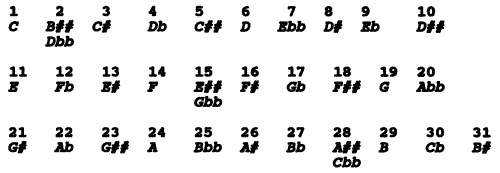```
```

## 5. Interval-invariancy: A general theory

There are several things worth noting about this system. Unlike the previous system, the note names are out of order. On the other hand, this system has the property that absolute pitch either stays the same or increases with each successive number. The octave is represented by 31 numbers, which leads to ambiguous representations for four of the 35 second-order pitches. The interesting question to ask is whether this representation system is an isolated case or whether it belongs to a class of systems, as the previous one did.

To answer this question, we need to develop a general theory for the construction of interval-invariant, number-line systems. The starting point for this theory is the recollection of the fact that all intervals found in common Western notation can be expressed as the sum or difference of m (a certain number of) fifths. A rising augmented second can be expressed as nine rising fifths and five falling octaves. Therefore, any number-line system for which fifths and octaves are invariant will be invariant for all other intervals as well.

Put another way, any system which is constructed by adding fifths together and folding the result around the octave will be an interval-invariant system. Such a construction is defined by two numbers: the number of units in a fifth, and the number of units in the octave. In theory, any two relatively prime, positive integers can be used to define such a construction and derive an interval-invariant system. However, to simplify the construction and the resulting system, the following conditions should also be imposed:

1. The number representing the octave should be equal to or greater than the number of distinct pitches to be represented in the octave. Choosing a smaller number will result in some ambiguities in the representation, as the Zimmerman system illustrates.
2. The number representing the fifth should ideally be more than half but less than two-thirds the number representing the octave. This condition is not strictly necessary, but without it systems may be constructed which spread the pitches within one octave over a range which exceeds the size of the octave interval.

With these conditions in place, we may approach the question of what mathematical properties are necessary to generate the two types of solutions we have studied so far. In the first class of solutions, which have the property that all pitches with the same note name are grouped together, the number representing C# is always one larger than the number representing C. Since the interval C C# can be expressed as seven rising fifths and four falling octaves, this condition may be expressed mathematically in the equation

```7 x A = (4 x B) + 1                                                     (1),
```
where A is the size of the fifth and B is the size of the octave. It can be shown that there is an infinite number of solutions which satisfy this condition. The set or class of solutions may be described as follows:
```The fifth = 7 + (4 x i); the octave = 12 + (7 x i), i = 0, 1, 2, ...    (2).
```

It is easy to verify that the solutions derived earlier, namely the 0-th order solution, the first-order solution, and the second-order solution, may be constructed from the number pairs <7,12> (i = 0), <15,26>(i = 2), and <23,40> (i = 4), respectively.

In the case of Zimmerman's system, the extra condition is that the number representing B# must always be one less than the number representing C. Since the interval B# - C can be expressed as seven rising octaves and twelve falling fifths, this condition may be expressed mathematically in the equation

```(7 x B) - (12 x A) = 1                                                  (3),
```

where A and B have the same meanings as in equation (1).

Zimmerman's system, in which A = 18 and B = 31, is one solution of this equation. Furthermore, it can be shown that this equation determines another class of solutions, described as follows:

```The fifth = 4 + (7 x i); the octave = 7 + (12 x i), i = 0, 1, 2 ...      (4).
```

Zimmerman's system corresponds to the solution where i = 2. For i = 3, we have the solution <25,43>, which is a true second-order solution of the Zimmerman type.

```
```

## 6. The base-40 system and the Clements system. Final propositions to the theory

In 1980, Peter Clements proposed a different interval-invariant number-line system for representing pitch notation. Clements began his construction by pointing out that all musical intervals in the diatonic system can be expressed as the sum of < x > (a certain number of) chromatic half steps and < y > (a certain number of) diatonic half steps. Clements based his construction on the principle that in most non-equal tempered tunings, the chromatic half step is slightly larger than the diatonic half step. Clements found that if he assigned the value of 80 to the diatonic half step and the value 88 to the chromatic half step, the resulting size of the octave (7 diatonic and 5 chromatic half steps) was exactly 1000. This system had the convenience that the thousands digit could be made to correspond directly with the octave number. If we divide the common factor of 8 out of all intervals, we end up with the generating pair (fifth = 73, octave = 125). This solution does not belong to either of the classes described above. In fact, if we follow Clements' procedure and impose the condition that the chromatic half step will always be one larger than the diatonic half step, mathematically expressed in the equation:

```(12 x A) - (7 x B) = 1                                            (5),
```
where A and B have the same meaning as in equation (1), then a new class of solutions emerges, which can be described as follows:
```The fifth = 3 + (7 x i); the octave = 5 + (12 x i), i  =  0, 1, 2, ...    (6).
```
The particular system devised by Clements corresponds to the value of i = 10.

With the number of "classes of solutions" now up to three, the reader may now be growing weary of the discussion of this problem. The ultimate question is whether or not there exists a simple way to describe all interval-invariant, number-line solutions, showing the classes of solutions described above as sub-groups of the whole. Using a combination of Clements' criterion, which describes all musical intervals as the sum or difference of diatonic and chromatic half steps, and the principle of generating representation systems from fifths and octaves as described above, it is possible to devise a classification system which covers all types of solutions (Ex. 8).

To complete the theory of this classification scheme, it should be pointed out that x and y can take on negative values. The solutions generated in this manner will fall outside the domain of practical usefulness, however.

This completes the theory of interval-invariant number-line systems suggested here.

```          Let x = size of the diatonic half step, and
let y = size of chromatic half step, where
x and y are positive integers.

Then a fifth = 4x + 3y and an octave = 7x + 5y

All solutions may now be plotted on the chart below, where the value of
x is posted along the horizontal dimension of the chart and the value of
y is posted along the vertical dimension of the chart.
```

Example 8:```
```

## Summary

The following important points are restated as a way of summarizing the contents of the approach:

1. Number-line representations of musical pitch notation have the advantage that information on pitch can be incorporated in one number.

2. Interval-invariant representation systems have the additional advantage that the difference between two pitch numbers will correctly determine the notated interval name between those pitches.

3. Each number-line representation system has a point beyond which the representation is ambiguous, if too many sharps or flats appear in front of a note. If the maximum number of sharps and flats is known in advance for a particular set of pitches, it is always possible to devise a number-line representation which will give unambiguous results for all pitches in the set.

4. Any number-line representation system which is constructed by adding and subtracting fifths and octaves of predetermined size will be interval-invariant. An interval-invariant representation is uniquely characterized by the size of the fifth and the size of the octave.

5. Franklin Zimmerman was the first person known to the present author to have devised and used an interval-invariant number-line representation system. His system, based on an octave size of 31, dates from 1975. In 1980, Peter Clements described a different system based on an octave size of 1000. The system presented in this paper was devised by the author in 1984. It is based on an octave size of 40 and is unambiguous for accidentals up to two sharps and two flats. Each of these systems is a member of a class of systems.

6. All interval-invariant representations may be classified according to Clements' criterion, which describes the size of the fifth and octave in terms of the size of the diatonic and chromatic half steps.
```
```

## References

```
Agmon, E. (1989).  "A Mathematical Model of the Diatonic System,"
Journal of Music Theory 33/1 (1989), 1-25.

Böker-Heil, N. (1971) "Ein algebraisches Modell des dur-moll-tonalen
Systems," In: Bericht über den 1.  Internationalen Kongress für
Musiktheorie Stuttgart 1971 (Stuttgart, 1971), 64-98.

Böker-Heil, N. (1984).  "Klingenden Dokumentationen aus dem Synthesizer,"
Musikdokumentation gestern, heute und morgen.  Harold Heckmann zum
60. Geburtstag am 6. Dezember 1984.  (Kassel, 1984), 19-24.

Bowles, G. H. (1978).  "Computer-Produced Thematic Catalogue: An Index
to the Pièces de violes of Marin Marais."  2 vols.  Ph.D. dissertation:
Stanford University

Brinkman, A. R. (1982).  "A Binomial Representation of the Pitch Parameter
for Computer Processing of Musical Data," Proceedings of the 1980
International Computer Music Conference, comp. Herbert S. Howe.
San Francisco, CA: Computer Music Assoc., 1982

Brinkman, A. R. (1986) "A Binomial Representation of Pitch for Computer
Processing of Musical Data," Music Theory Spectrum Vol. 8 (1986), 44-57.

Clements, P. J. (1982).  "Musical Data Structures in a Multi-use
Environment."  Proceedings of the 1980 International Computer Music
Conference, comp. Herbert S. Howe.  San Francisco, CA: Computer Music
Assoc., 1982.

Zimmerman, F. B. (1975).  Henry Purcell, 1659 - 1695: Melodic and Interval
Indexes to his Complete Works. Philadelphia: Smith-Edwards-Dunlap.

```
```

```